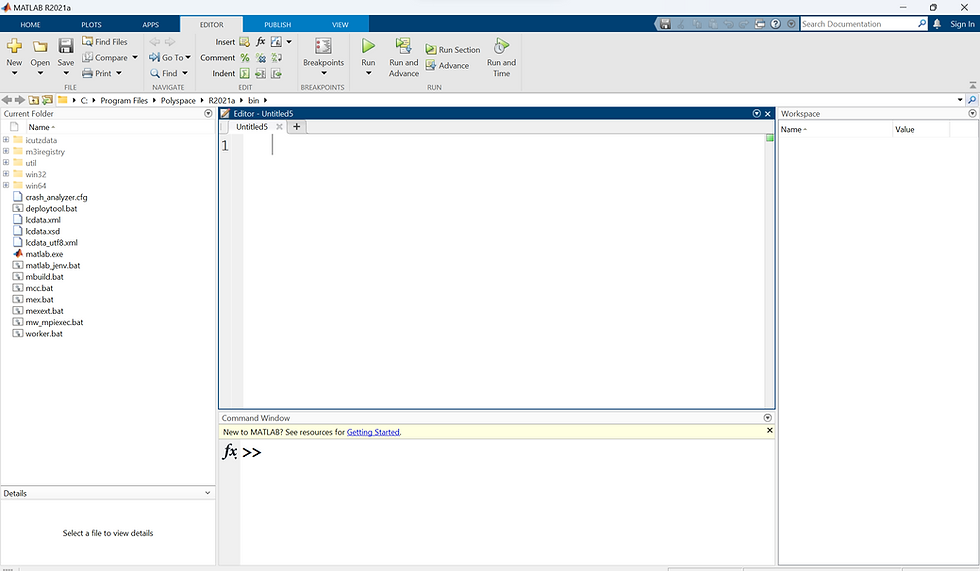top of page

# "MATLAB for Beginners: A Quick Introduction to a Powerful Tool"

Updated: Aug 29

MATLAB is a powerful software that has become increasingly popular over the years. It is a programming language that is commonly used in engineering, science, and mathematics. If you are new to MATLAB, it may seem overwhelming at first. However, with a little bit of effort, you can become proficient in using this software. In this blog, we will discuss the basics of MATLAB and provide some tips on how to get started.

Firstly, let's define what MATLAB is. MATLAB stands for Matrix Laboratory. As the name suggests, it is designed to work with matrices. Matrices are essentially arrays of numbers, and MATLAB is particularly well-suited for manipulating and analyzing matrices. MATLAB is widely used in a variety of fields, such as signal processing, control systems, and image processing, to name just a few.The MATLAB software can be downloaded from the MathWorks website, and it comes with a user-friendly graphical user interface (GUI). The GUI makes it easy to create and edit scripts and functions, and it provides access to a wide range of built-in functions and toolboxes. MATLAB also has an active user community, and there are many resources available online to help you learn how to use it.

To get started with MATLAB, the first thing you should do is become familiar with the MATLAB workspace. The workspace is where all the variables and data are stored when you are working in MATLAB. To view the workspace, you can use the command "whos" in the command window. The command window is where you can enter commands and run scripts. You can also use the workspace to view and edit variables directly.Next, you should become familiar with basic MATLAB syntax. MATLAB syntax is similar to other programming languages, and it uses keywords, variables, and functions to manipulate data. MATLAB uses parentheses to enclose arguments for functions and square brackets to create matrices. For example, to create a matrix in MATLAB, you can use the following syntax:

```
A = [1 2 3; 4 5 6; 7 8 9];

```

This creates a 3x3 matrix called A with values 1 through 9.

MATLAB is based on matrices and arrays, and almost all variables are matrices or arrays of some sort. You can create a matrix by enclosing its elements in square brackets and separating them by commas or spaces. For example, to create a 3x3 matrix with random values, you can type:

```
A = rand(3,3)

```This will create a matrix A with random values between 0 and 1.

MATLAB provides a wide range of functions for working with matrices and arrays. For example, you can perform basic arithmetic operations on matrices, such as addition, subtraction, multiplication, and division. You can also perform more advanced operations, such as matrix inversion, eigen decomposition, and singular value decomposition.

Once you are comfortable with basic syntax, you can begin exploring the built-in functions and toolboxes that are available in MATLAB. MATLAB has a wide range of functions and toolboxes for everything from signal processing to machine learning. You can access these functions and toolboxes by typing the function or toolbox name in the command window, or by using the GUI.

In addition to built-in functions, you can also create your own functions in MATLAB. Functions are useful for encapsulating complex operations and for reusing code. To create a function in MATLAB, you can use the following syntax:

```
function [output1,output2,...] = myFunction(input1,input2,...)
% Function body
end```This creates a function called "myFunction" that takes inputs "input1" and "input2" and returns outputs "output1" and "output2".

MATLAB also provides a range of plotting functions that allow you to create high-quality 2D and 3D plots of your data. For example, to plot a sine wave, you can type:

```
t = linspace(0,2*pi,100);
y = sin(t);
plot(t,y);

```

This will create a plot of a sine wave over the interval [0,2π] with 100 data points.Finally, it is important to remember that MATLAB is a powerful tool, and it can take some time to become proficient in using it. However, with practice and patience, you can become a skilled MATLAB user.

In conclusion, MATLAB is a powerful software that is commonly used in engineering, science, and mathematics. If you are new to MATLAB, this blog post provides a basic introduction to its functionality and capabilities. As you continue to use MATLAB, you will discover more of its features and become more proficient in its use.